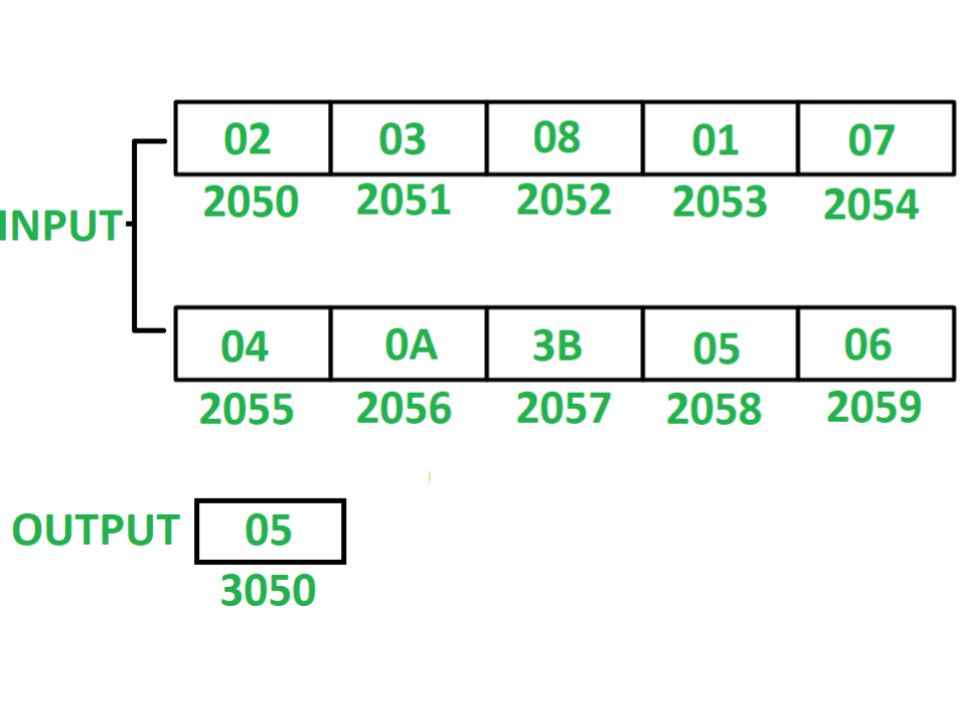# 8085 program to count total even numbers in series of 10 numbers

Program – Write an assembly language program in 8085 microprocessor to count even numbers in series of 10 numbers.

Example –Assumption – Ten 8-bit numbers are stored from starting memory location 2050. Value of count is stored at memory location 3050.

Algorithm –

1. Initialize register H with 20 and register L with 4F so that indirect memory points to memory location 204F.
2. Initialize register C with 00 and register D with 0A.
3. Increment indirect memory by 1.
4. Store value of M in accumulator A.
5. Check whether the content in A is even or odd by performing AND operation of A with 01.
6. If content of A is 00 after AND operation then number scanned was even, If so then increment C by 01 else if content of A is 01 after AND operation then number scanned was odd, If so then decrements D by 01.
7. Check if zero flag is not set i.e. ZF = 0 then jump to step 3 otherwise store value of C at memory location 3050.

Program –

2000 LXI H 204F H <- 20, L <- 4F
2003 MVI C, 00 C <- 00
2005 MVI D, 0A D <- 0A
2007 INX H M <- M + 01
2008 MOV A, M A <- M
2009 ANI 01 A <- A (AND) 01
200B JNZ 200F Jump if ZF = 0
200E INR C C <- C + 01
200F DCR D D <- D – 01
2010 JNZ 2007 Jump if ZF = 0
2013 MOV A, C A <- C
2014 STA 3050 M <- A
2017 HLT END

Explanation – Registers A, B, C, D, H, L are used for general purpose.

1. LXI H 204F: assign 20 to H and 4F to L.
2. MVI C, 00: assign 00 to C.
3. MVI D, 0A: assign 0A to D.
4. INX H: increment indirect memory location M by 01.
5. MOV A, M: move content of M to A.
6. ANI 01: perform AND operation of A with 01 and store the result in A.
7. JNZ 200F: jump if ZF = 0 to memory location 200F.
8. INR C: increment C by 01.
9. DCR D: decrements D by 01.
10. JNZ 2007: jump if ZF = 0 to memory location 2007.
11. MOV A, C: moves the content of C to A.
12. STA 3050: store the content of A to memory location 3050.
13. HLT: stops executing the program and halts any further execution.

My Personal Notes arrow_drop_upCheck out this Author's contributed articles.

If you like GeeksforGeeks and would like to contribute, you can also write an article using contribute.geeksforgeeks.org or mail your article to contribute@geeksforgeeks.org. See your article appearing on the GeeksforGeeks main page and help other Geeks.

Please Improve this article if you find anything incorrect by clicking on the "Improve Article" button below.